CBSE Class 12 Sample Paper for 2018 Boards

Class 12
Solutions of Sample Papers and Past Year Papers - for Class 12 Boards

### If the function f : R → R be defined by f(x) = 2x − 3 and g : R → R by g(x) = x 3 + 5, then find fog and show that  fog  is invertible. Also, find ( fog ) −1 , hence find ( fog ) −1 (9).

This is a question of CBSE Sample Paper - Class 12 - 2017/18.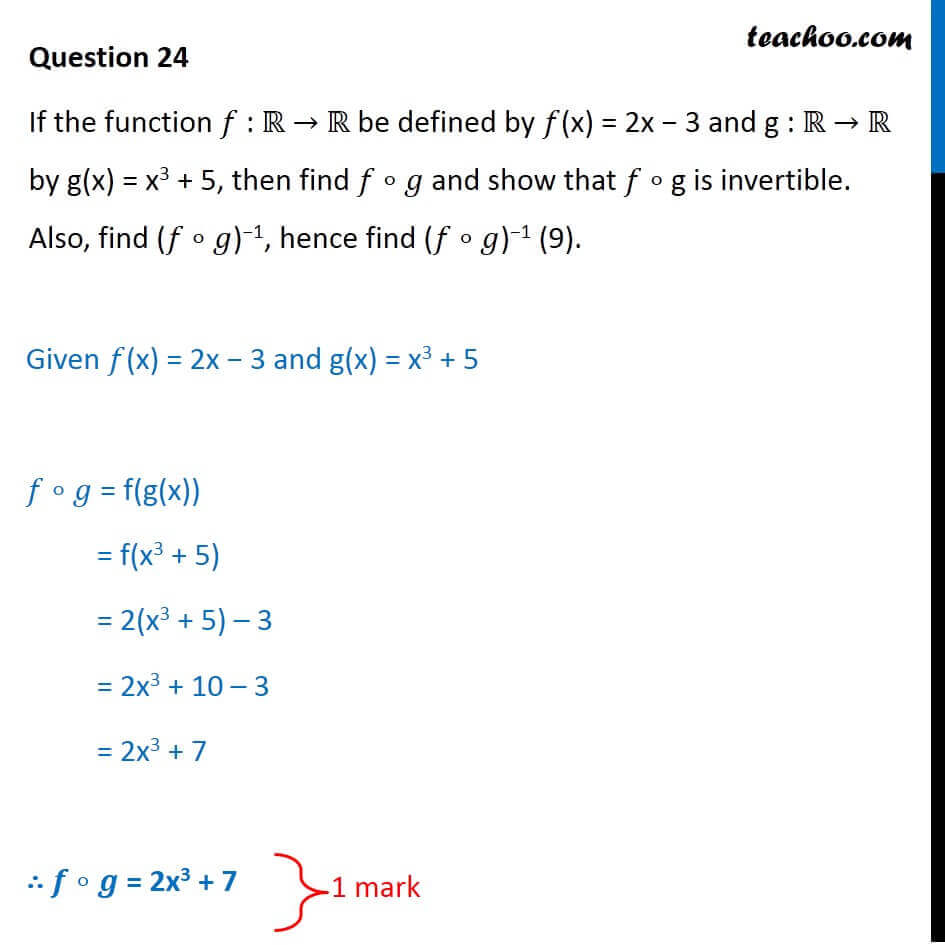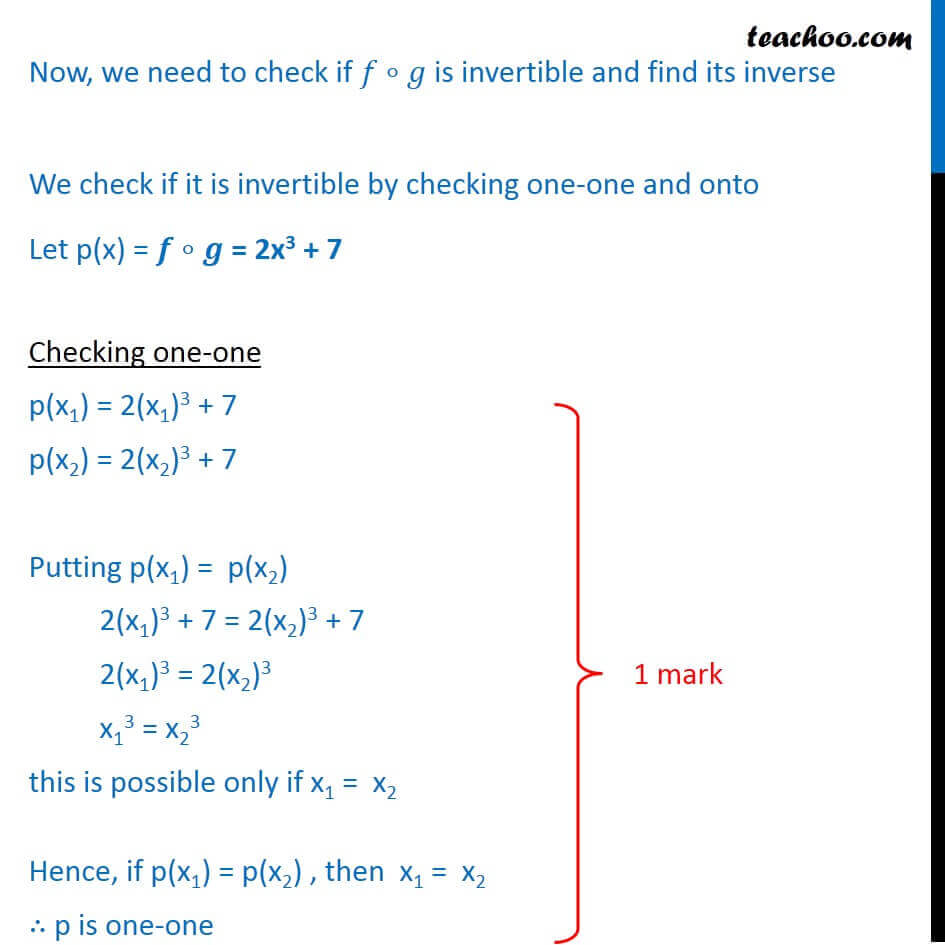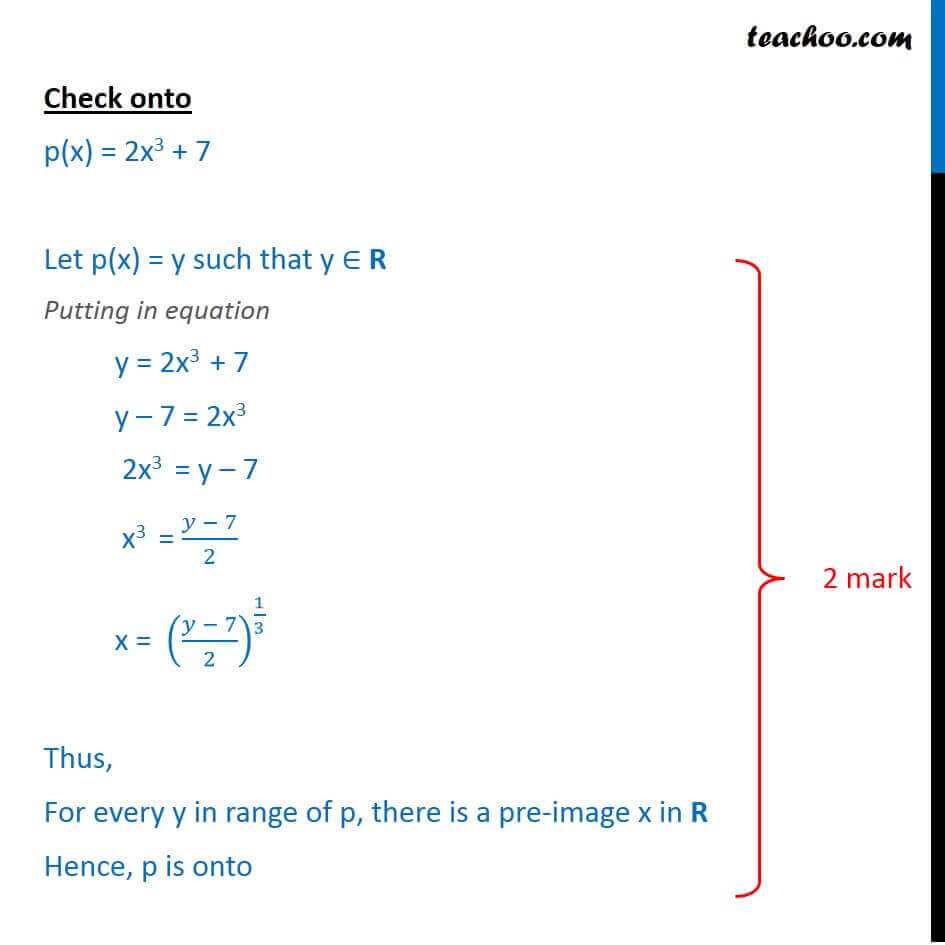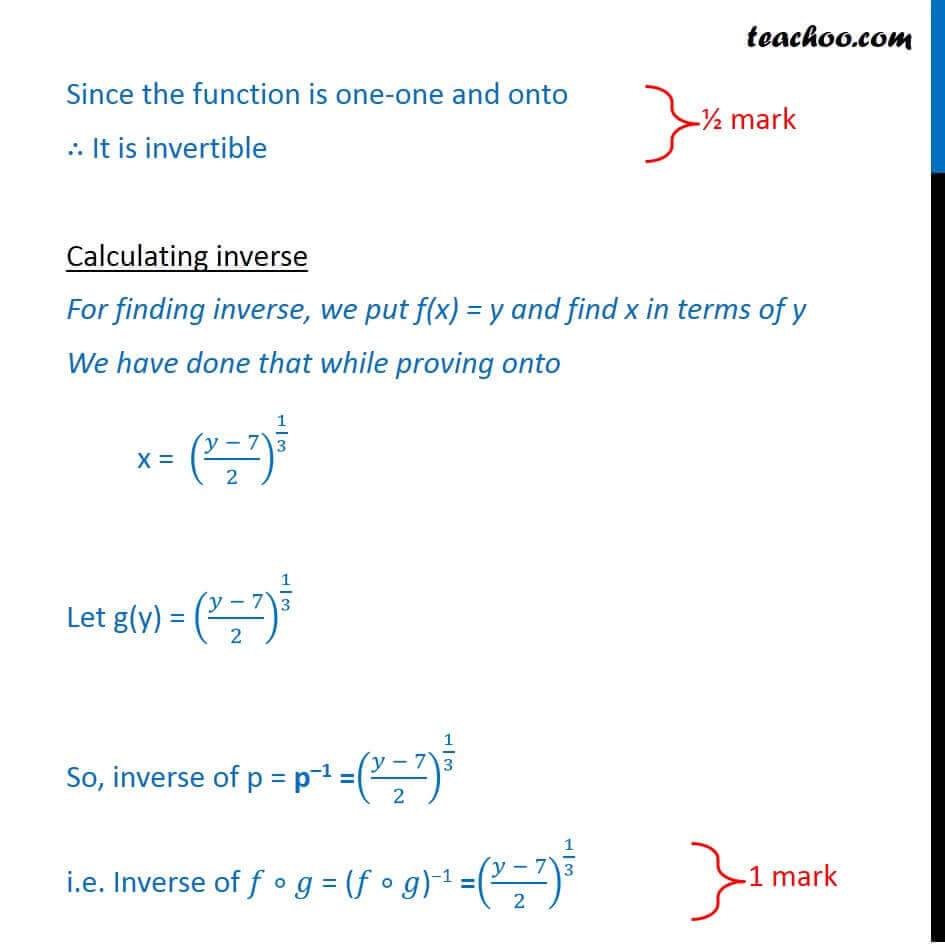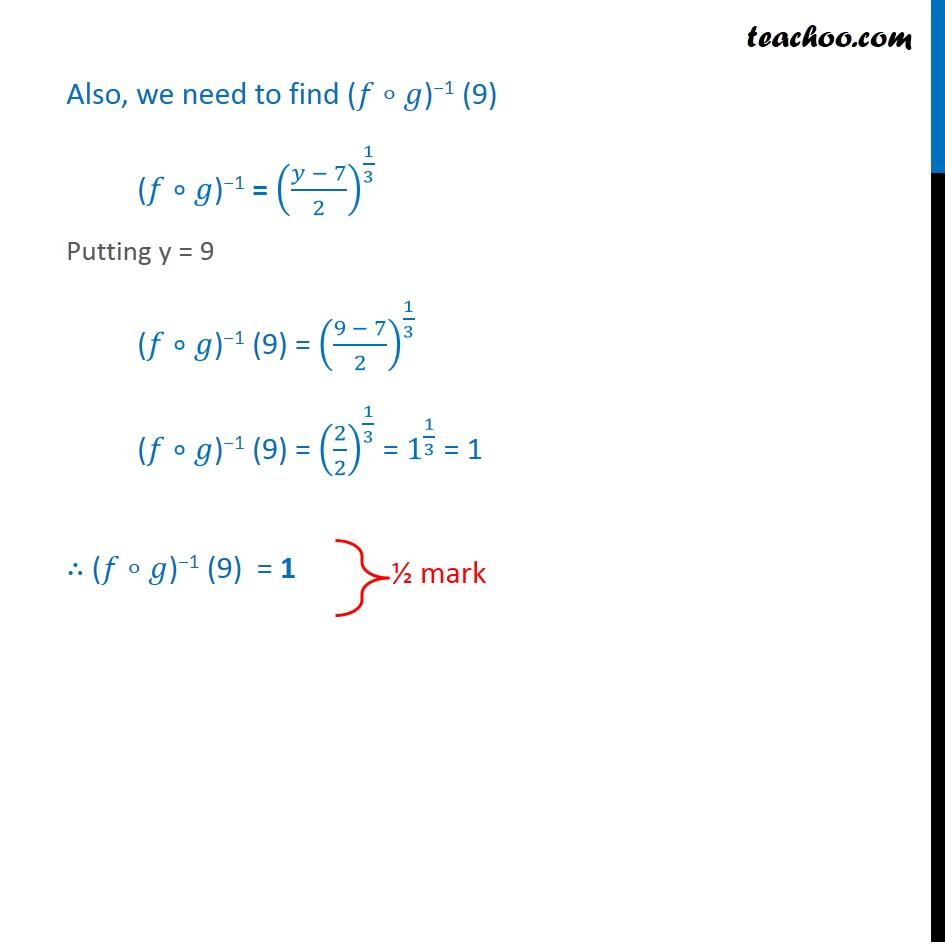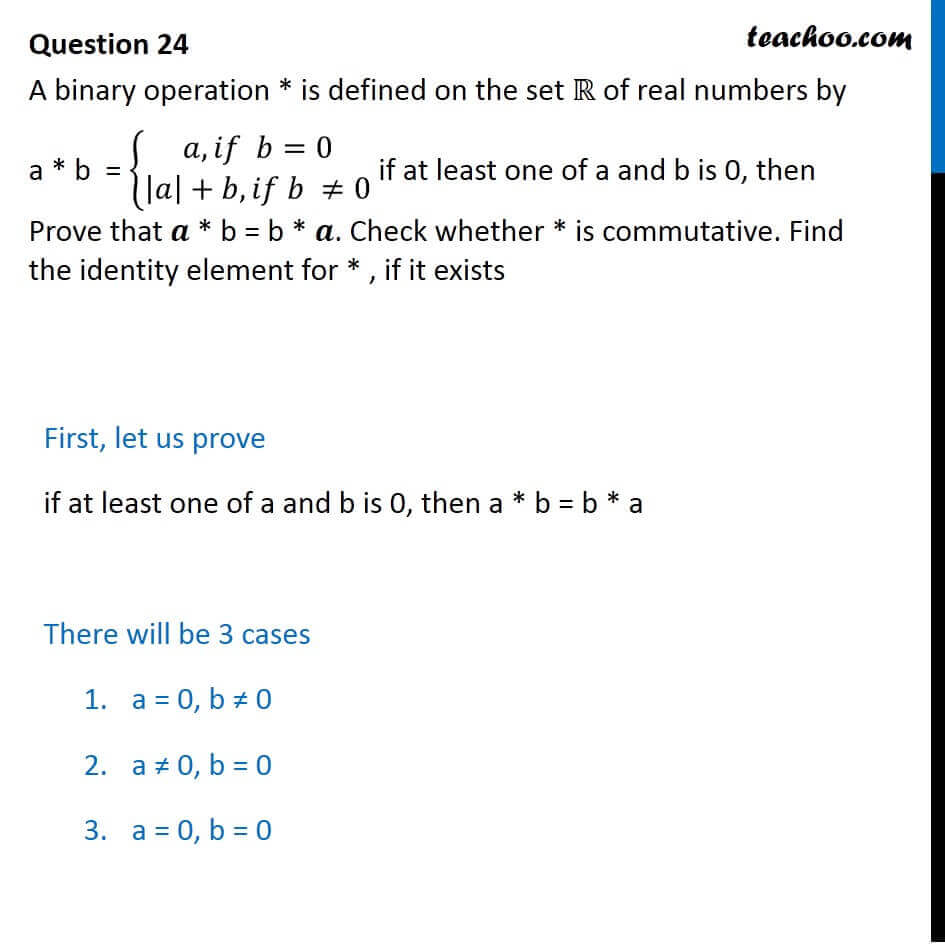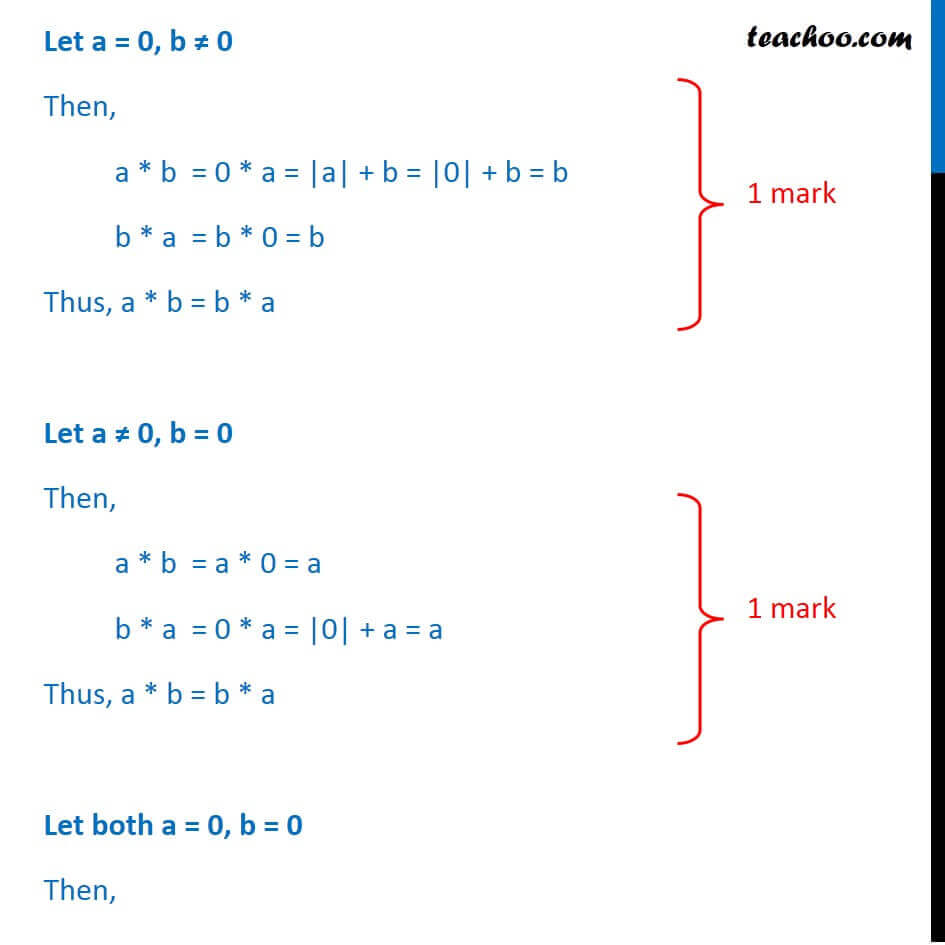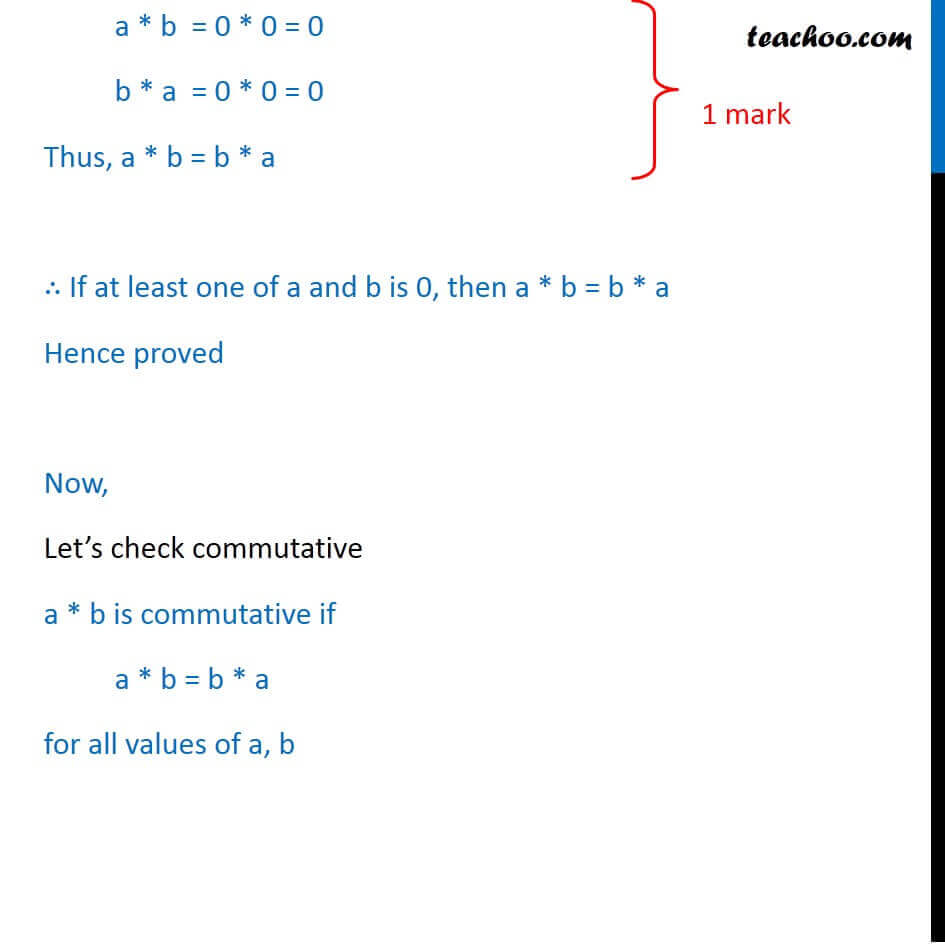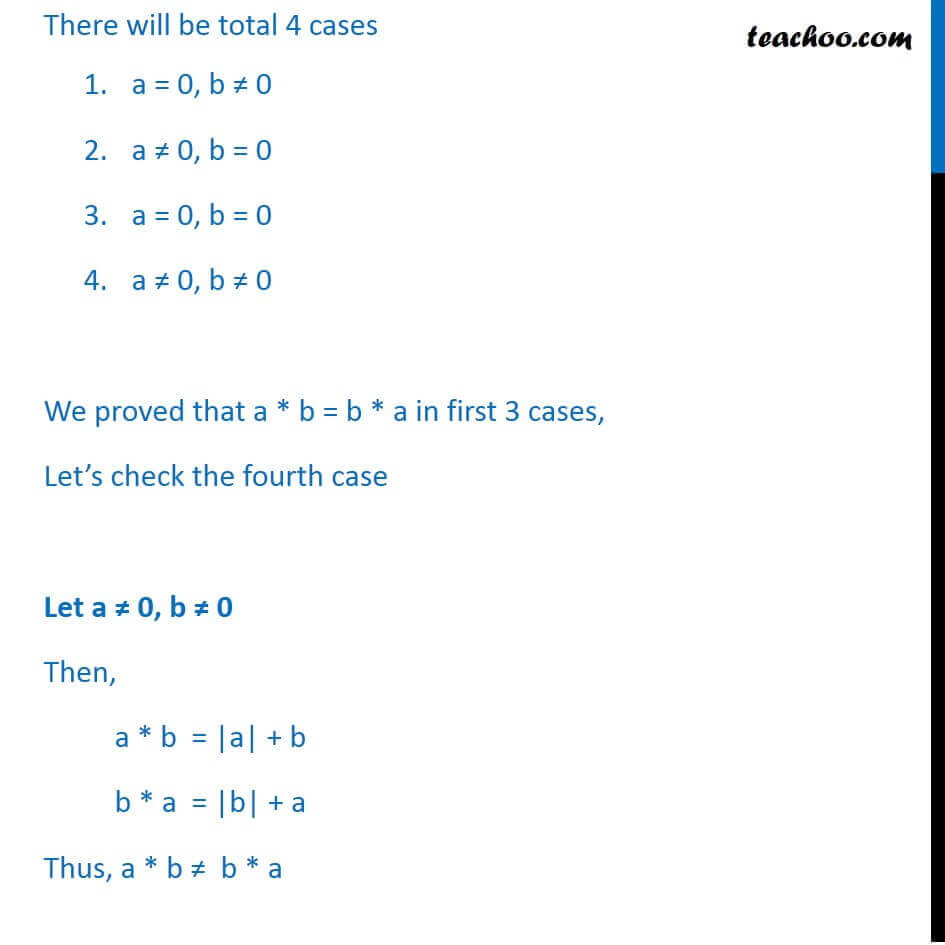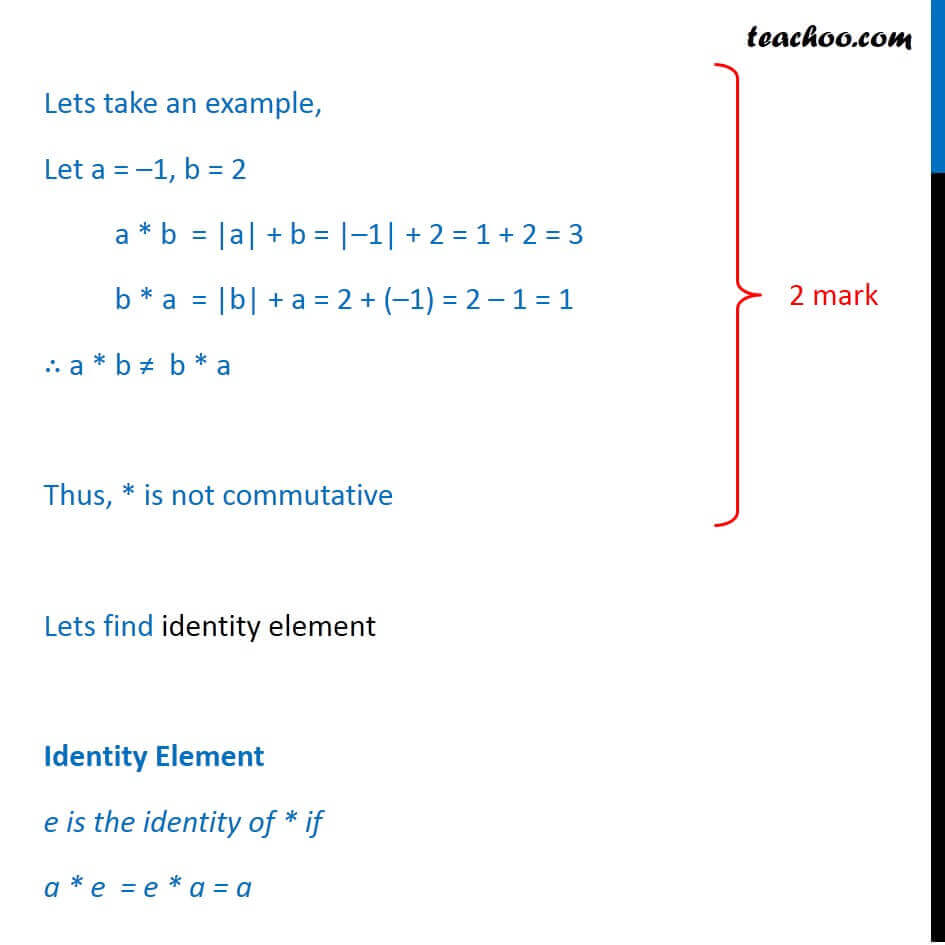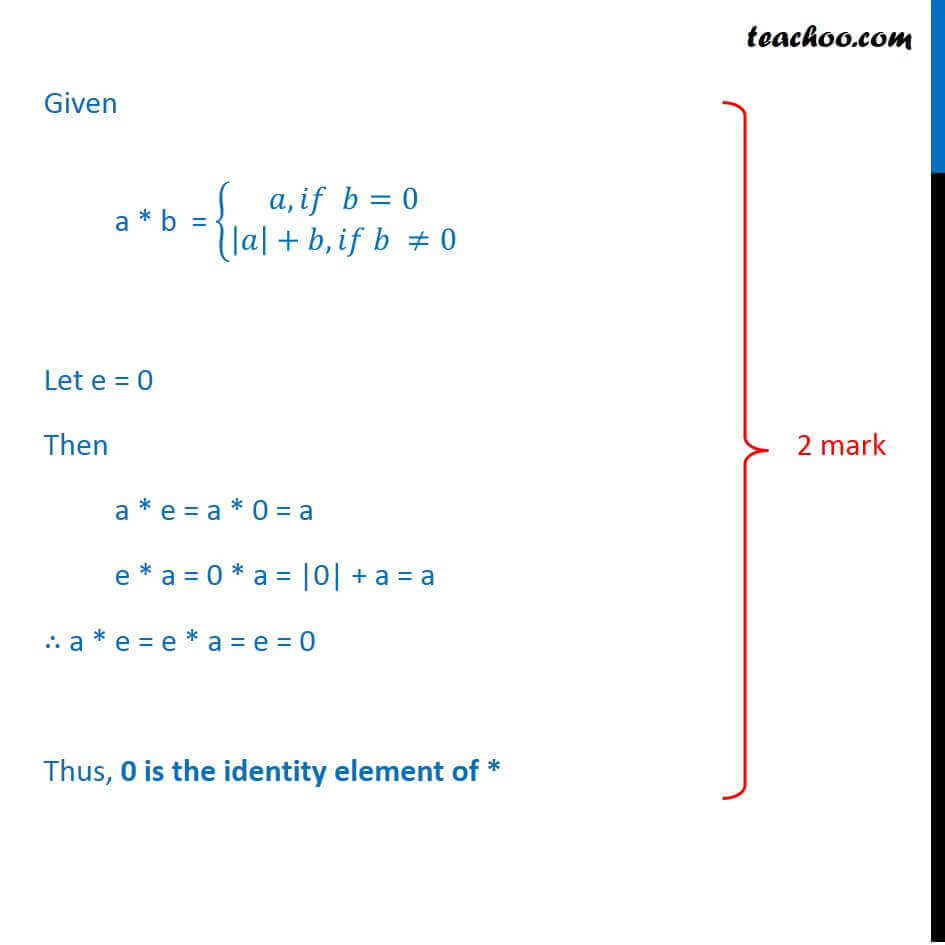Learn in your speed, with individual attention - Teachoo Maths 1-on-1 Class

### Transcript

Question 24Question 24 A binary operation * is defined on the set ℝ of real numbers by a * b = {█(𝑎, 𝑖𝑓 𝑏=0@|𝑎|+𝑏, 𝑖𝑓 𝑏 ≠0)┤ if at least one of a and b is 0, then Prove that 𝒂 * b = b * 𝒂. Check whether * is commutative. Find the identity element for * , if it exists If the function 𝑓 : ℝ → ℝ be defined by 𝑓(x) = 2x − 3 and g : ℝ → ℝ by g(x) = x3 + 5, then find 𝑓∘𝑔 and show that 𝑓 ∘ g is invertible. Also, find (𝑓∘𝑔)−1, hence find (𝑓∘𝑔)−1 (9). Given 𝑓(x) = 2x − 3 and g(x) = x3 + 5 𝑓∘𝑔 = f(g(x)) = f(x3 + 5) = 2(x3 + 5) – 3 = 2x3 + 10 – 3 = 2x3 + 7 ∴ 𝒇∘𝒈 = 2x3 + 7 Now, we need to check if 𝑓∘𝑔 is invertible and find its inverse We check if it is invertible by checking one-one and onto Let p(x) = 𝒇∘𝒈 = 2x3 + 7 Checking one-one p(x1) = 2(x1)3 + 7 p(x2) = 2(x2)3 + 7 Putting p(x1) = p(x2) 2(x1)3 + 7 = 2(x2)3 + 7 2(x1)3 = 2(x2)3 x13 = x23 this is possible only if x1 = x2 Hence, if p(x1) = p(x2) , then x1 = x2 ∴ p is one-one Check onto p(x) = 2x3 + 7 Let p(x) = y such that y ∈ R Putting in equation y = 2x3 + 7 y – 7 = 2x3 2x3 = y – 7 x3 = (𝑦 − 7)/2 x = ((𝑦 − 7)/2)^(1/3) Thus, For every y in range of p, there is a pre-image x in R Hence, f is onto Since the function is one-one and onto ∴ It is invertible Calculating inverse For finding inverse, we put f(x) = y and find x in terms of y We have done that while proving onto x = ((𝑦 − 7)/2)^(1/3) Let g(y) = ((𝑦 − 7)/2)^(1/3) So, inverse of p = p–1 =((𝑦 − 7)/2)^(1/3) i.e. Inverse of 𝑓∘𝑔 = (𝑓∘𝑔)−1 =((𝑦 − 7)/2)^(1/3) Also, we need to find (𝑓∘𝑔)−1 (9) (𝑓∘𝑔)−1 = ((𝑦 − 7)/2)^(1/3) Putting y = 9 (𝑓∘𝑔)−1 (9) = ((9 − 7)/2)^(1/3) (𝑓∘𝑔)−1 (9) = (2/2)^(1/3) = 1^(1/3) = 1 ∴ (𝑓∘𝑔)−1 (9) = 1 First, let us prove if at least one of a and b is 0, then a * b = b * a There will be 3 cases a = 0, b ≠ 0 a ≠ 0, b = 0 a = 0, b = 0 Let a = 0, b ≠ 0 Then, a * b = 0 * a = |a| + b = |0| + b = b b * a = b * 0 = b Thus, a * b = b * a Let a ≠ 0, b = 0 Then, a * b = a * 0 = a b * a = 0 * a = |0| + a = a Thus, a * b = b * a Let both a = 0, b = 0 Then, a * b = 0 * 0 = 0 b * a = 0 * 0 = 0 Thus, a * b = b * a ∴ If at least one of a and b is 0, then a * b = b * a Hence proved Now, Let’s check commutative a * b is commutative if a * b = b * a for all values of a, b a * b = 0 * 0 = 0 b * a = 0 * 0 = 0 Thus, a * b = b * a ∴ If at least one of a and b is 0, then a * b = b * a Hence proved Now, Let’s check commutative a * b is commutative if a * b = b * a for all values of a, b There will be total 4 cases a = 0, b ≠ 0 a ≠ 0, b = 0 a = 0, b = 0 a ≠ 0, b ≠ 0 We proved that a * b = b * a in first 3 cases, Let’s check the fourth case Let a ≠ 0, b ≠ 0 Then, a * b = |a| + b b * a = |b| + a Thus, a * b ≠ b * a There will be total 4 cases a = 0, b ≠ 0 a ≠ 0, b = 0 a = 0, b = 0 a ≠ 0, b ≠ 0 We proved that a * b = b * a in first 3 cases, Let’s check the fourth case Let a ≠ 0, b ≠ 0 Then, a * b = |a| + b b * a = |b| + a Thus, a * b ≠ b * a Lets take an example, Let a = –1, b = 2 a * b = |a| + b = |–1| + 2 = 1 + 2 = 3 b * a = |b| + a = 2 + (–1) = 2 – 1 = 1 ∴ a * b ≠ b * a Thus, * is not commutative Lets find identity element Identity Element e is the identity of * if a * e = e * a = a Given a * b = {█(𝑎, 𝑖𝑓 𝑏=0@|𝑎|+𝑏, 𝑖𝑓 𝑏 ≠0)┤ Let e = 0 Then a * e = a * 0 = a e * a = 0 * a = |0| + a = a ∴ a * e = e * a = e = 0 Thus, 0 is the identity element of *